﻿ 变系数异方差模型的贝叶斯分析

# 变系数异方差模型的贝叶斯分析Bayesian Analysis of Varying Coefficient Heteroscedastic Models

Abstract: Based on variance modeling, Bayesian estimation and outlier identification of varying coefficient heteroscedastic models are studied, where the nonparametric part is approximated by B-spline. By combining the Gibbs sampler and Metropolis-Hastings algorithm, Bayesian estimation and Bayesian diagnosis statistics based on the K-L distance are obtained to identify outliers. Simulation studies show that the proposed Bayesian methods are feasible and effective.

1. 引言

2. 模型与符号

2.1. 基于方差建模的变系数异方差模型

$\left\{\begin{array}{l}{y}_{i}={x}_{i}^{\text{T}}\beta +{z}_{i}^{\text{T}}\alpha \left({u}_{i}\right)+{\epsilon }_{i},{\epsilon }_{i}~N\left(0,{\sigma }_{i}^{2}\right),\\ {\sigma }_{i}^{2}=g\left({h}_{i}^{\text{T}}\gamma \right),\\ i=1,2,\cdots ,n.\end{array}$ (1)

$L\left(\beta ,\gamma ,\alpha \left(\cdot \right)|Y,X,Z,H,U\right)={\left(2\pi \right)}^{-\frac{n}{2}}\underset{i=1}{\overset{n}{\prod }}{\left({\sigma }_{i}^{2}\right)}^{-\frac{1}{2}}\mathrm{exp}\left(-\frac{1}{2}\underset{i=1}{\overset{n}{\sum }}\frac{{\left({y}_{i}-{x}_{i}^{\text{T}}\beta -{z}_{i}^{\text{T}}\alpha \left({u}_{i}\right)\right)}^{2}}{{\sigma }_{i}^{2}}\right)$ (2)

${\mu }_{i}={x}_{i}^{\text{T}}\beta +{B}_{i}^{\text{T}}\lambda$

$L\left(\theta |Y,X,Z,H,U\right)={\left(2\pi \right)}^{-\frac{n}{2}}\underset{i=1}{\overset{n}{\prod }}{\left({\sigma }_{i}^{2}\right)}^{-\frac{1}{2}}\mathrm{exp}\left(\frac{1}{2}\underset{i=1}{\overset{n}{\sum }}\frac{{\left({y}_{i}-{x}_{i}^{\text{T}}\beta -{B}_{i}^{\text{T}}\lambda \right)}^{2}}{{\sigma }_{i}^{2}}\right)$(3)

2.2. K-L距离

K-L距离也称作K-L信息，具有距离和信息的某些性质，在统计上以反映两个模型或分布的差异而著称。根据韦博成  对K-L距离的介绍，密度函数为 $f\left(x\right)$$g\left(x\right)$ 的两个分布的K-L距离 $K\left(f,g\right)$ 被定义为： $K\left(f,g\right)={E}_{f}\left\{\mathrm{log}\frac{f\left(x\right)}{g\left(x\right)}\right\}$，其中 ${E}_{f}$ 表示对 $f\left(x\right)$ 求期望。

3. 贝叶斯分析

3.1. 先验分布

3.2. Gibbs抽样和条件分布

· 抽样 ${\tau }^{2\left(l+1\right)}$

$p\left({\tau }^{2}|\lambda \right)\propto {\left({\tau }^{2}\right)}^{-\frac{K}{2}-{a}_{\tau }-1}\mathrm{exp}\left\{\frac{-\frac{1}{2}{\left(\lambda -{\lambda }_{0}\right)}^{\text{T}}\left(\lambda -{\lambda }_{0}\right)-{b}_{\tau }}{{\tau }^{2}}\right\}$ (4)

· 抽样 ${\lambda }^{\left(l+1\right)}$

$p\left(\lambda |Y,X,Z,H,U,\beta ,\gamma \right)\propto \mathrm{exp}\left\{-\frac{1}{2}{\left(\lambda -{\lambda }^{*}\right)}^{\text{T}}{\Sigma }_{\lambda }^{\ast -1}\left(\lambda -{\lambda }^{*}\right)\right\}$(5)

· 抽样 ${\beta }^{\left(l+1\right)}$

$p\left(\beta |Y,X,Z,H,U,\gamma ,\lambda \right)\propto \mathrm{exp}\left\{-\frac{1}{2}{\left(\beta -{b}^{*}\right)}^{\text{T}}{B}^{*-1}\left(\beta -{b}^{*}\right)\right\}$(6)

· 抽样 ${\gamma }^{\left(l+1\right)}$

$p\left(\gamma |Y,X,Z,H,U,\beta ,\lambda \right)\propto \mathrm{exp}\left\{-\frac{1}{2}\underset{i=1}{\overset{n}{\sum }}{h}_{i}^{\text{T}}\gamma -\frac{1}{2}\underset{i=1}{\overset{n}{\sum }}\frac{{\left({y}_{i}-{x}_{i}^{\text{T}}\beta -{B}_{i}^{\text{T}}\lambda \right)}^{2}}{\mathrm{exp}\left({h}_{i}^{\text{T}}\gamma \right)}-\frac{1}{2}{\left(\gamma -{\gamma }_{0}\right)}^{\text{T}}{\sum }_{\gamma }^{-1}\left(\gamma -{\gamma }_{0}\right)\right\}$ (7)

3.3. 贝叶斯估计

$\stackrel{^}{\beta }=\frac{1}{J}\underset{j=1}{\overset{J}{\sum }}{\beta }^{\left(j\right)},\stackrel{^}{\gamma }=\frac{1}{J}\underset{j=1}{\overset{J}{\sum }}{\gamma }^{\left(j\right)},\stackrel{^}{\lambda }=\frac{1}{J}\underset{j=1}{\overset{J}{\sum }}{\lambda }^{\left(j\right)}$

$Var\left(\theta |Y,X,Z,H,U\right)={\left(J-1\right)}^{-1}\underset{j=1}{\overset{J}{\sum }}\left({\theta }^{\left(j\right)}-\stackrel{^}{\theta }\right){\left({\theta }^{\left(j\right)}-\stackrel{^}{\theta }\right)}^{\text{T}}$

3.4. 贝叶斯诊断

$K\left(P,{P}_{-i}\right)=\int p\left(\theta |D\right)\mathrm{log}\left\{\frac{p\left(\theta |D\right)}{p\left(\theta |{D}_{-i}\right)}\right\}\text{d}\theta$(8)

$K\left(P,{P}_{-i}\right)=\mathrm{log}{E}_{\theta }\left[\frac{L\left(\theta |{D}_{-i}\right)}{L\left(\theta |D\right)}|D\right]+{E}_{\theta }\left[\mathrm{log}\frac{L\left(\theta |D\right)}{L\left(\theta |{D}_{-i}\right)}|D\right]$(9)

$K\left(P,{P}_{-i}\right)=\mathrm{log}\left[\frac{1}{J}\underset{j=1}{\overset{J}{\sum }}\frac{L\left({\theta }^{\left(j\right)}|{D}_{-i}\right)}{L\left({\theta }^{\left(j\right)}|D\right)}\right]+\frac{1}{J}\underset{j=1}{\overset{J}{\sum }}\mathrm{log}\left[\frac{L\left({\theta }^{\left(j\right)}|D\right)}{L\left({\theta }^{\left(j\right)}|{D}_{-i}\right)}\right]$(10)

4. 模拟研究

TypeI：

${\beta }_{0}={\left(1,-0.5,0.5\right)}^{\text{T}},{\sum }_{\beta }=0.25×{Ι}_{\text{3}},{\gamma }_{0}={\left(1,-0.5,0.5\right)}^{\text{T}},{\sum }_{\gamma }=0.25×{Ι}_{3},{\lambda }_{0}={\left(0,\cdots ,0\right)}^{\text{T}},{a}_{\tau }={b}_{\tau }=1$。这种设置表示具有很好的先验信息。

TypeII：

${\beta }_{0}=3×{\left(1,-0.5,0.5\right)}^{\text{T}},{\sum }_{\beta }={Ι}_{\text{3}},{\gamma }_{0}=3×{\left(1,-0.5,0.5\right)}^{\text{T}},{\sum }_{\gamma }={Ι}_{3},{\lambda }_{0}={\left(0,\cdots ,0\right)}^{\text{T}},{a}_{\tau }={b}_{\tau }=1$。这种设置表示具有较差的先验信息。

TypeIII：

${\beta }_{0}={\left(0,0,0\right)}^{\text{T}},{\sum }_{\beta }=10×{Ι}_{3},{\gamma }_{0}={\left(0,0,0\right)}^{\text{T}},{\sum }_{\gamma }=10×{Ι}_{3},{\lambda }_{0}={\left(0,\cdots ,0\right)}^{\text{T}},{a}_{\tau }={b}_{\tau }=1$。这些超参数值的设置代表的是没有先验信息的情况。Table 1. Bayesian estimation of model parameters under different sample sizes and prior distributionsFigure 1. The average estimated curves of nonparametric parts ${\alpha }_{1}\left(u\right)$ and ${\alpha }_{2}\left(u\right)$ when n = 80 and prior information of Type I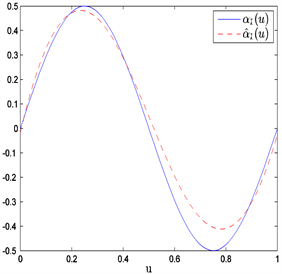Figure 2. The average estimated curves of nonparametric parts ${\alpha }_{1}\left(u\right)$ and ${\alpha }_{2}\left(u\right)$ when n = 80 and prior information of Type II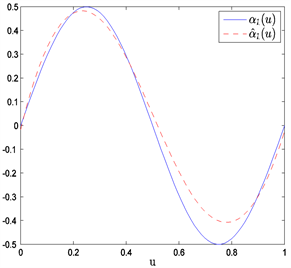Figure 3. The average estimated curves of nonparametric parts ${\alpha }_{1}\left(u\right)$ and ${\alpha }_{2}\left(u\right)$ when n = 80 and prior information of Type III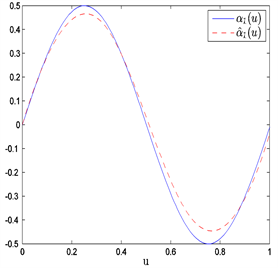Figure 4. The average estimated curves of nonparametric parts ${\alpha }_{1}\left(u\right)$ and ${\alpha }_{2}\left(u\right)$ when n = 150 and prior information of Type I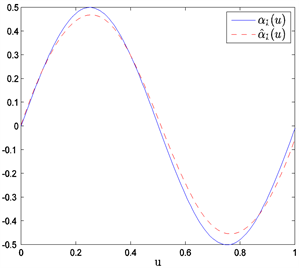Figure 5. The average estimated curves of nonparametric parts ${\alpha }_{1}\left(u\right)$ and ${\alpha }_{2}\left(u\right)$ when n = 150 and prior information of Type II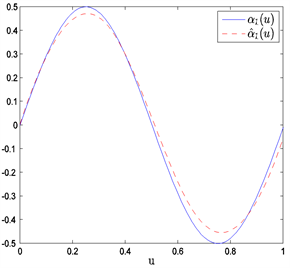Figure 6. The average estimated curves of nonparametric parts ${\alpha }_{1}\left(u\right)$ and ${\alpha }_{2}\left(u\right)$ when n = 150 and prior information of Type III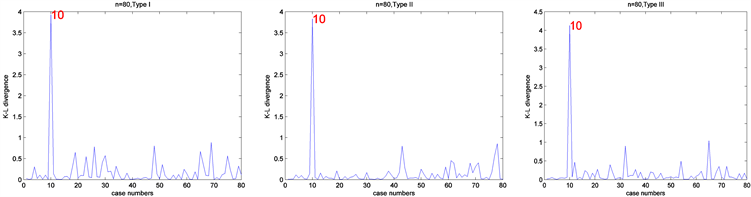Figure 7. Bayesian case deletion diagnosis results based on different prior information and n = 80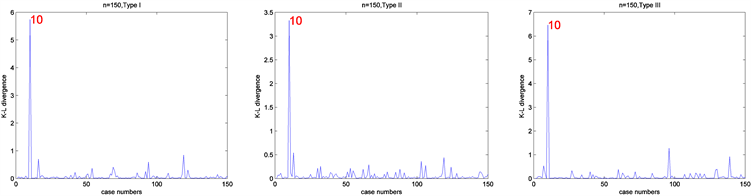Figure 8. Bayesian case deletion diagnosis results based on different prior information and n = 150

5. 结论

 吴刘仓, 张忠占, 徐登可. 联合均值与方差模型的变量选择[J]. 系统工程理论与实践, 2012(8): 1754-1760.

 李双双, 吴刘仓, 戴琳. 联合均值与方差混合专家回归模型的参数估计[J]. 应用数学, 2019, 32(1): 134-140.

 赵远英, 吴刘仓, 徐登可. 带有不可忽略缺失数据的联合均值与方差模型的贝叶斯估计[J]. 昆明理工大学学报(自然科学版), 2020, 45(1): 125-132.

 戴琳, 陶治, 吴刘仓. 联合均值与方差模型的统计诊断[J]. 统计与信息论坛, 2017, 32(1): 14-19.

 赵远英, 徐登可, 庞一成. 联合均值与方差模型的Bayes分析[J]. 高校应用数学学报, 2018, 33(2): 241-252.

 Cook, R.D. (1977) Detection of Influential Observations in Linear Regression. Technometrics, 19, 15-18.
https://doi.org/10.1080/00401706.1977.10489493

 Cho, H., Ibrahim, J.G., Sinha, D. and Zhu, H.T. (2009) Bayesian Case Influence Diagnostics for Survival Models. Biometrics, 65, 116-124.
https://doi.org/10.1111/j.1541-0420.2008.01037.x

 Tang, N.S. and Duan, X.D. (2012) A Semiparametric Bayesian Approach to Generalized Partial Linear Mixed Models for Longitudinal Data. Computational Statistics & Data Analysis, 56, 4348-4365.
https://doi.org/10.1016/j.csda.2012.03.018

 He, X.M. and Shi, P. (1994) Convergence Rate of B-Spline Estimators of Nonparametric Conditional Quantile Function. Journal of Nonparametric Statistics, 3, 299-308.
https://doi.org/10.1080/10485259408832589

 韦博成. 参数统计教程[M]. 北京: 高等教育出版社, 2006.

 Geyer, C.J. (1992) Practical Markov Chain Monte Carlo. Statistical Science, 7, 473-511.
https://doi.org/10.1214/ss/1177011137

Top